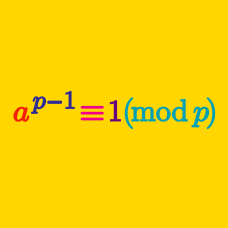Number Theory

# Modular Arithmetic - Word Problems

There are $81$ groups of $21$ students. When we regroup all of the students so that each group has $5$ members, how many students will be left without a group?

John and Amy have $48$ and $51$ quarters, respectively. If they combine all of their quarters and change as much as possible into dollar bills, how many quarters will be left?

Note: There are 4 quarters in a dollar.

November and December have $30$ and $31$ days, respectively. If November $1$ is the first day of the week, how many days of December are there in the last week?

How many different values are there for $4 ^ n \pmod { 7}$, as $n$ ranges over all positive integers?

What is the sum of all positive prime numbers $x$ such that $x^2+2$ is also a prime?

×